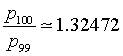The search for a sequence of natural numbers such that the ratio between each of them and the previous one converges to the plastic number was also made by the architect Richard Padovan who discovered that the sequence pn generated by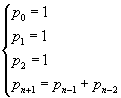has the same behavior as the Perrin sequence. This sequence is said Padovan sequence and its terms Padovan numbers.

Padovan numbers pi can be deduced as follows.

1. Let pi bi a linear combination of the i-th powers of the roots α, β and γ of the equation x3-x-1 = 0, where α is real and β and γ conjugate complex numbers with absolute value < 1: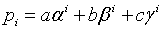2. Let p0, p1 and p2 be equal to 1: you have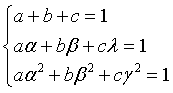3. The solution of the system is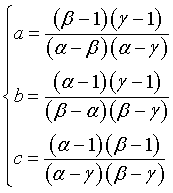4. so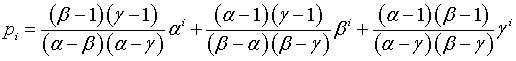This definition of pi implies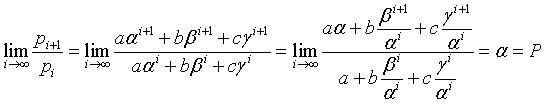because as the exponent approaches the infinity, the powers of β and γ converge to 0.

Moreover you have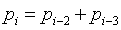that is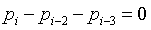Infact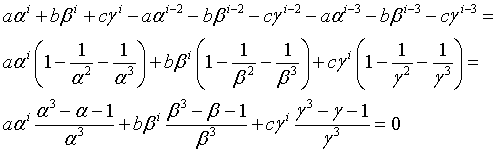The use of the recursive definition for the computer calculation of the n-th Padovan number is impractical. Better to use a loop, as in the following Javascript implementation

```function nPadovan(n)
{
n = parseInt(n);
if (n < 2) return 1;
var n1 = 1;
var n2 = 1;
var n3 = 1;
var p;
for (var i=3; i<=n; i++)
{
p = n2 + n3;
n3 = n2;
n2 = n1;
n1 = p;
}
return(p);
}
```

index i

number

If you can use Mathematica (Wolfram), you may define the following function

```PadovanN[n_]:=Switch[n,0,1,1,1,2,1,_,Module[{i,n1,n2,n3,val},n1=1;n2=1;n3=1;
For[i=3,i <= n,i++,val=n2+n3;n3=n2;n2=n1;n1=val];val]]
{1,1,1,2,2,3,4,5,7,9,12,16,21,28,37,49,65,86,114,151,200,265,351,465,616,816,1081,1432,1897,2513,3329}
```

In general, any sequence of numbers qi expressed as a linear combination with coefficients a, b, c of the i-th powers of the roots α, β and γ, given three arbitrary values for q0 , q1 and q2, shows the same recursion and the ratio between each term and the previous one converges to P.

For example, the sequence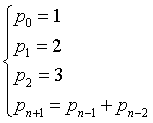that is

 1 2 3 3 5 6 8 11 14 19 25 33 44 58 77 102 135 179 237 ...

is such that